Bayesian Statistical Methods

Partial solutions

Chapter 1: Basics of Bayesian Inference

Jump to probem: 1, 3, 5, 7, 9, 11, 13, 15, 17

(1) The integral over the PDF is $$\int_{1}^{\infty} c\exp(-x/\theta)dx = c\theta\exp(-1/\theta)$$ and therefore if $$c=\exp(1/\theta)/\theta$$ the PDF is valid.

(3) Since the parameter must be positive we select a Gamma$$(a,b)$$ prior. Setting the mean and variance of the prior to the desired mean and variance gives $a/b = 5 \mbox{ and }a/b^2=3.$ Solving for $$a$$ and $$b$$ gives $$a=25/3$$ and $$b=5/3$$. We can check these answers using MC sampling:

x  <- rgamma(10000000,25/3,5/3)
mean(x);var(x)
##  4.999478
##  2.997442

(5a) The marginal PDF is \begin{align} f(x_1) &= \int f(x_1,x_2)dx_2\\ &\propto \int \exp\left[-\frac{x_1^2-2\rho x_1x_2+x_2^2}{2(1-\rho^2)}\right] dx_2\\ &\propto \exp\left[-\frac{x_1^2}{2(1-\rho^2)}\right] \int \exp\left[-\frac{-2\rho x_1x_2+x_2^2}{2(1-\rho^2)}\right] dx_2\\ &\propto \exp\left[-\frac{x_1^2 - \rho^2x_1^2}{2(1-\rho^2)}\right] \int \exp\left[-\frac{\rho^2x_1^2-2\rho x_1x_2+x_2^2}{2(1-\rho^2)}\right] dx_2\\ &\propto \exp\left[-\frac{x_1^2}{2}\right] \int \exp\left[-\frac{(x_2-\rho x_1)^2}{2(1-\rho^2)}\right] dx_2\\ &\propto \exp\left[-\frac{x_1^2}{2}\right], \end{align} and therefore $$X_1$$ is standard normal.

(5b) Before computing the conditional distribution, we note if $$Y\sim\mbox{Normal}(\mu,\sigma^2)$$ then $f(y) \propto \exp\left[-\frac{y^2-2\mu y + \mu^2}{2\sigma^2}\right]\propto\exp[-(\tau y^2 -2y\tau\mu)/2],$ where $$\tau=1/\sigma^2$$. Therefore, to find the conditional distribution we will rearrange the PDF to have this form and solve for $$\mu$$ and $$\tau$$. \begin{align} f(x_1|x_2) &= f(x_1,x_2)/f(x_2)\\ &\propto \exp\left[-\frac{x_1^2-2\rho x_1x_2+x_2^2}{2(1-\rho^2)}\right]\\ &\propto \exp\left[-\frac{x_1^2-2\rho x_1x_2}{2(1-\rho^2)}\right]\\ &\propto \exp\left[-(\tau x_1^2-2x_1\tau\mu)/2\right],\\ \end{align} where $$\tau=1/(1-\rho^2)$$ and $$\mu=\rho x_2$$. Therefore, $$X_1|X_2\sim\mbox{Normal}(\rho X_2,1-\rho^2)$$.

(7) First, the overall probability of a car being stolen ($$R$$ = Raleigh, $$D$$ = Durham) is \begin{align} \mbox{Prob}(\mbox{stolen}) &= \mbox{Prob}(\mbox{stolen}|R)\mbox{Prob}( R)+ \mbox{Prob}(\mbox{stolen}|D)\mbox{Prob}(D)\\ & = \frac{135}{10000}\frac{2}{3} + \frac{214}{10000}\frac{1}{3} = 0.01613333. \end{align} Applying Bayes' rule, \begin{align} \mbox{Prob}(R|\mbox{stolen}) &= \frac{\mbox{Prob}(\mbox{stolen}|R)\mbox{Prob}( R)}{\mbox{Prob}(\mbox{stolen})}\\ &= \frac{\frac{135}{10000}\frac{2}{3}}{0.01613333} = 0.5578512. \end{align}

(9) The data for each word is the number of keystroke errors and the likelihood is Binomial$$(3,\theta)$$. Therefore, the data are

words = c("fun","sun","sit","sat","fan","for")
e_sun = c(1,0,2,2,2,3)
e_the = c(3,3,3,3,3,3)
e_foo = c(2,3,3,3,2,1)

These vectors give the number of errors between each typed word and each word in the dictionary.

(9a)

alpha = 2; theta <- 0.1

prior = ifelse(words=="for",alpha,1)
prior = prior/sum(prior)
names(prior) <- words
round(prior,2)
##  fun  sun  sit  sat  fan  for
## 0.14 0.14 0.14 0.14 0.14 0.29
like_sun = (theta^e_sun)*((1-theta)^(3-e_sun))
post_sun = like_sun*prior/sum(like_sun*prior)
names(post_sun) <- words
round(post_sun,2)
##  fun  sun  sit  sat  fan  for
## 0.10 0.87 0.01 0.01 0.01 0.00
like_the = (theta^e_the)*((1-theta)^(3-e_the))
post_the = like_the*prior/sum(like_the*prior)
names(post_the) <- words
round(post_the,2)
##  fun  sun  sit  sat  fan  for
## 0.14 0.14 0.14 0.14 0.14 0.29
like_foo = (theta^e_foo)*((1-theta)^(3-e_foo))
post_foo = like_foo*prior/sum(like_foo*prior)
names(post_foo) <- words
round(post_foo,2)
##  fun  sun  sit  sat  fan  for
## 0.05 0.01 0.01 0.01 0.05 0.89

(9b)

alpha = 50; theta <- 0.1

prior = ifelse(words=="for",alpha,1)
prior = prior/sum(prior)
names(prior) <- words
round(prior,2)
##  fun  sun  sit  sat  fan  for
## 0.02 0.02 0.02 0.02 0.02 0.91
like_sun = (theta^e_sun)*((1-theta)^(3-e_sun))
post_sun = like_sun*prior/sum(like_sun*prior)
names(post_sun) <- words
round(post_sun,2)
##  fun  sun  sit  sat  fan  for
## 0.09 0.82 0.01 0.01 0.01 0.06
like_the = (theta^e_the)*((1-theta)^(3-e_the))
post_the = like_the*prior/sum(like_the*prior)
names(post_the) <- words
round(post_the,2)
##  fun  sun  sit  sat  fan  for
## 0.02 0.02 0.02 0.02 0.02 0.91
like_foo = (theta^e_foo)*((1-theta)^(3-e_foo))
post_foo = like_foo*prior/sum(like_foo*prior)
names(post_foo) <- words
round(post_foo,2)
##  fun  sun  sit  sat  fan  for
## 0.00 0.00 0.00 0.00 0.00 0.99

When $$\alpha$$ increases all the probabilities on “for'' increase.

(9c)

alpha = 2; theta <- 0.95

prior = ifelse(words=="for",alpha,1)
prior = prior/sum(prior)
names(prior) <- words
round(prior,2)
##  fun  sun  sit  sat  fan  for
## 0.14 0.14 0.14 0.14 0.14 0.29
like_sun = (theta^e_sun)*((1-theta)^(3-e_sun))
post_sun = like_sun*prior/sum(like_sun*prior)
names(post_sun) <- words
round(post_sun,2)
##  fun  sun  sit  sat  fan  for
## 0.00 0.00 0.02 0.02 0.02 0.93
like_the = (theta^e_the)*((1-theta)^(3-e_the))
post_the = like_the*prior/sum(like_the*prior)
names(post_the) <- words
round(post_the,2)
##  fun  sun  sit  sat  fan  for
## 0.14 0.14 0.14 0.14 0.14 0.29
like_foo = (theta^e_foo)*((1-theta)^(3-e_foo))
post_foo = like_foo*prior/sum(like_foo*prior)
names(post_foo) <- words
round(post_foo,2)
##  fun  sun  sit  sat  fan  for
## 0.02 0.32 0.32 0.32 0.02 0.00

When $$\theta$$ is greater than a half, errors are more likely than not, and so words with many errors have higher probability.

(11) We will plot the posterior on a grid of $$\lambda$$ from 0 to 100 and then zoomed in around 20 to 40.

lambda <- seq(0,100,0.01)       # A grid for plotting
Y      <- c(64,13,33,18,30,20)  # The data
prior  <- dunif(lambda,0,100)
like   <- 1
for(t in 1:6){like<-like*dpois(Y[t],lambda)}
post   <- like*prior/sum(like*prior)

plot(lambda,post,xlab=expression(lambda),
ylab="Posterior",type="l")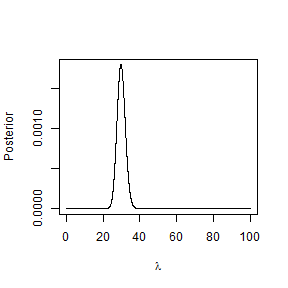plot(lambda,post,xlab=expression(lambda),
ylab="Posterior",type="l",xlim=c(20,40))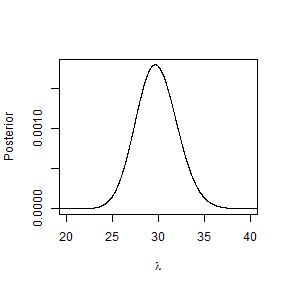(13a)

a1 <- 25
b1 <- 10
a2 <- 10
b2 <- 15

Y  <- seq(0.001,0.999,0.001)
d1 <- dbeta(Y,a1,b1)
d2 <- dbeta(Y,a2,b2)
d3 <- 0.9*d1 + 0.1*d2
plot(Y,d1,type="l",col=3,xlab="y",ylab="Density")
lines(Y,d2,col=2)
lines(Y,d3,col=1)
legend("topleft",c("Forest","Deforest","Mixture"),
lty=1,col=3:1,bty="n")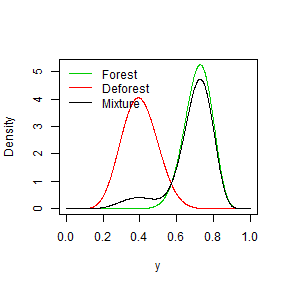plot(Y,d3,type="l",xlab="y",ylab="Mixture density")
abline(v=0.458,lty=2)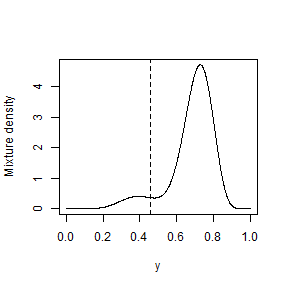(13b) Let $$d_k(y)$$ be the beta density of $$y=NDVI$$ for forested ($$k=1$$) and deforested ($$k=2$$) pixels. The expression is
$\mbox{Prob}(\mbox{Deforested}|Y=y) = \frac{d_2(y)*0.1}{d_1(y)*0.9+d_2(y)*0.1}.$

post <- d2*0.1/(d1*0.9+d2*0.1)
plot(Y,post,type="l",xlab="NDVI",
ylab="Posterior probability deforested")
abline(0.9,0,lty=2)
abline(v=0.458,lty=2)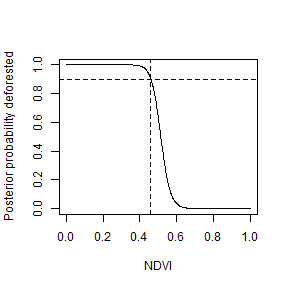(13c) The rule is to conclude the pixel is deforested if NDVI $$<$$ 0.458 (as shown in the last plot of part a).

(15a) We observe $$n=10$$, $$Y=2$$ and fit the model $$Y|\theta\sim\mbox{Binomial}(n,\theta)$$. The prior is $$\theta\sim\mbox{Beta}(1,1)$$ (i.e., uniform) and so the posterior is $$\theta|Y\sim\mbox{Beta}(A,B),$$ where $$A=Y+1=3$$ and $$B=n-Y+1=9$$. The posterior mean and SD are

A <- 3
B <- 9

A/(A+B)                       # Posterior mean
##  0.25
sqrt(A*B)/((A+B)*sqrt(A+B+1)) # Posterior SD
##  0.1200961
# Verify the calculations with MC sampling
theta <- rbeta(100000,A,B)
mean(theta);sd(theta)
##  0.2498564
##  0.1203142

(15b) The HPD interval is computed by searching over quantiles of the form $$(\tau,0.95+\tau)$$ for $$\tau\in(0,0.05)$$ for the shortest interval.

q1 <- qbeta(c(0.025,0.975),A,B) # equal-tailed
q1
##  0.06021773 0.51775585
tau   <- seq(0.00,0.05,0.001)
width <- qbeta(0.95+tau,A,B)-qbeta(tau,A,B)
plot(tau,width,type="l")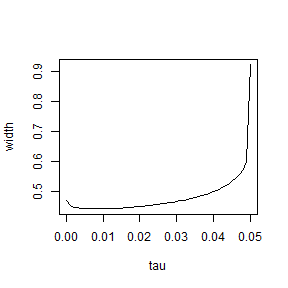tau   <- tau[which.min(width)]
tau
##  0.009
q2 <- qbeta(c(tau,0.95+tau),A,B) # HPD
q2
##  0.04120976 0.48438956
theta<-seq(0,1,0.001)
plot(theta,dbeta(theta,A,B),type="l",
xlab=expression(theta),ylab="Posterior PDF")
abline(v=q1,col=3)
abline(v=q1,col=3)
abline(v=q2,col=4)
abline(v=q2,col=4)
legend("topright",c("Equal-tailed","HPD"),
col=3:4,lty=1,bty="n")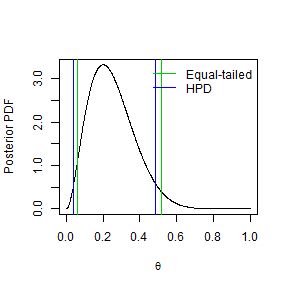(15c) The probability is approximated using Monte Carlo sampling.

N     <- 1000000            # Number of MC samples
theta <- rbeta(N,A,B)       # Samples from posterior
Y     <- rbinom(N,10,theta) # Samples from PPD
plot(table(Y)/N,xlab="Y",ylab="PPD")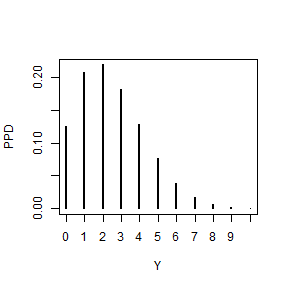mean(Y>0)                   # Prob(Y-star>0|Y=2)
##  0.87547

(17a) Let $$Y_i$$ and $$n_i$$ be the number of made and attempted clutch shots for player $$i$$. The model is $$Y_i|\theta_i\sim\mbox{Binomial}(n_i,\theta_i)$$ and the uninformative prior is $$\theta_i\sim\mbox{Beta}(1,1)$$. The posterior is then $$\theta_i|Y_i\sim\mbox{Beta}(Y_i+1,n_i-Y_i+1)$$.

(17b) The data are:

Y      <- c(64,72,55,27,75,24,28,66,40,13)
n      <- c(75,95,63,39,83,26,41,82,54,16)
q      <- c(0.845,0.847,0.880,0.674,0.909,
0.898,0.770,0.801,0.802,0.875)

The posterior densities are:

theta <- seq(0,1,0.001)
plot(NA,xlim=c(0.4,1),ylim=c(0,12),
xlab=expression(theta),ylab="Posterior density")
for(i in 1:10){
lines(theta,dbeta(theta,Y[i]+1,n[i]-Y[i]+1),col=i)
}
legend("topleft",player,lty=1,col=1:10,bty="n",ncol=2)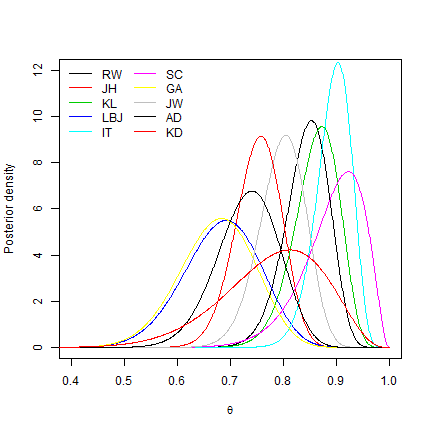(17c) The table below gives the posterior median and 95% interval for each player

q50    <- qbeta(0.500,Y+1,n-Y+1)
q_low  <- qbeta(0.025,Y+1,n-Y+1)
q_high <- qbeta(0.975,Y+1,n-Y+1)

out    <- round(cbind(q50,q_low,q_high),2)
rownames(out) <- player
kable(out)
q50 q_low q_high
RW 0.85 0.76 0.92
JH 0.75 0.66 0.83
KL 0.87 0.77 0.93
LBJ 0.69 0.53 0.81
IT 0.90 0.82 0.95
SC 0.90 0.76 0.98
GA 0.68 0.53 0.80
JW 0.80 0.71 0.88
AD 0.73 0.61 0.84
KD 0.79 0.57 0.93

(17d) To determine if the percentages are different we compute the $\mbox{Prob}(\theta_i < q_i|Y_i)$ where $$q_i$$ is the non-clutch proportion. If this probability is close to zero or one then we can conclude there is a difference.

prob        <- pbeta(q,Y+1,n-Y+1)
names(prob) <- player
round(prob,2)
##   RW   JH   KL  LBJ   IT   SC   GA   JW   AD   KD
## 0.48 0.99 0.64 0.44 0.64 0.47 0.92 0.51 0.89 0.85

It looks like James Harden is worse in the clutch.

(17e) Below we compare the previous results from a Beta(1,1) prior with those from Beta(0.5,0.5) and Beta(2,2).

prob05        <- pbeta(q,Y+0.5,n-Y+0.5)
names(prob05) <- player
prob2         <- pbeta(q,Y+2,n-Y+2)
names(prob2)  <- player
round(prob,2)
##   RW   JH   KL  LBJ   IT   SC   GA   JW   AD   KD
## 0.48 0.99 0.64 0.44 0.64 0.47 0.92 0.51 0.89 0.85
round(prob05,2)
##   RW   JH   KL  LBJ   IT   SC   GA   JW   AD   KD
## 0.44 0.99 0.59 0.41 0.59 0.37 0.90 0.48 0.87 0.79
round(prob2,2)
##   RW   JH   KL  LBJ   IT   SC   GA   JW   AD   KD
## 0.57 0.99 0.74 0.48 0.74 0.66 0.94 0.57 0.92 0.92

The probabilities change a bit so the results are somewhat sensitive to the prior. In all cases though we would conclude that only James Harden has a different percentage in the two settings.